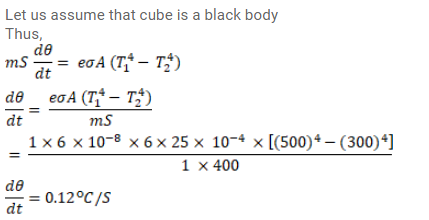# A cubical block of mass 1.0 kg and edge 5.0 cm`
Question:

A cubical block of mass $1.0 \mathrm{~kg}$ and edge $5.0 \mathrm{~cm}$ is heated to $227^{\circ} \mathrm{C}$ it is kept in an evacuated chamber maintained at $27^{\circ} \mathrm{C}$ Assuming that the block emits radiation like a blackbody, find the rate at which the temperature of the block will decrease. Specific heat capacity of the material of the block is $400 \mathrm{~J} / \mathrm{kg}-\mathrm{K}$.

Solution: